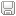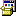#### sort 사용하기

아이템

```class SubtitleItem
{
public:
enum SUBTITLE_DIRECTION { LEFT, RIGHT };

float time;
SUBTITLE_DIRECTION direction;
ofImage* image;

static bool comp(const SubtitleItem *t1, const SubtitleItem *t2) { // 중요!
return (t1->time < t2->time);
}
};```

활용

```cout << "SORT TEST" << endl;
cout << "=========== before =========" << endl;

for (int i = 0; i < collection.size(); i++)
{
cout << collection[i]->time << endl;
}

//sort
sort(collection.begin(), collection.end(), SubtitleItem::comp);

cout << "============ after =========" << endl;
for (int i = 0; i < collection.size(); i++)
{
cout << collection[i]->time << endl;
}```

결과#### 'C++(일반)' 카테고리의 다른 글

 sort 사용하기  (0) 2016.08.19 2016.08.10 2016.03.27 2016.03.27 2016.03.25 2016.03.25
2016. 8. 19. 15:25

### 댓글을 달아 주세요

#### Cmd 명령으로 프로그램 실행 하기

C#의 Process.Start 처럼 ,

C++ 에서도 가능하다.

아래의 예제는 ffmpeg.exe 를 통해 웹에서 재생이 가능한 영상으로 변환 하는 옵션을 포함 하고 있다.

프로세스를 마칠때 까지 해당 쓰레드는 멈춘다.

```LPWSTR ConvertToLPWSTR(const std::string& s)
{
LPWSTR ws = new wchar_t[s.size() + 1]; // +1 for zero at the end
copy(s.begin(), s.end(), ws);
ws[s.size()] = 0; // zero at the end
return ws;
}

...

cout << "start" << endl;

SHELLEXECUTEINFO processInfo;
ZeroMemory(&processInfo , sizeof(processInfo));
processInfo.cbSize = sizeof(processInfo);

processInfo.lpVerb = ConvertToLPWSTR("open");
processInfo.lpFile = ConvertToLPWSTR("ffmpeg.exe");
processInfo.lpParameters = ConvertToLPWSTR("-y -i capture.wmv -vcodec libx264 -f mp4 -b:v 2048k -r 30 x_wmv_win_mpeg.mp4");

processInfo.nShow = SW_HIDE; // SW_SHOW

int r = (int)ShellExecuteEx(&processInfo);
WaitForSingleObject(processInfo.hProcess, INFINITE); // 프로세스가 끝날때까지 기다림

cout << "end : " << r << endl;
```

#### 'C++(일반)' 카테고리의 다른 글

 sort 사용하기  (0) 2016.08.19 2016.08.10 2016.03.27 2016.03.27 2016.03.25 2016.03.25
2016. 8. 10. 13:38

### 댓글을 달아 주세요

#### _memcpy(...) with start Index.

```int len = 10;
int dst[] = { -1,-1,-1,-1,-1,-1,-1,-1,-1,-1 };
int src[] = {  0 ,1 ,2 ,3 ,4 ,5 ,6 ,7 ,8 ,9 };

int sp = 5; // 시작 인덱스
int read_count = len - sp; // 카운트
_memccpy(&dst[sp], &src[sp], 1 , read_count * sizeof(int));

for (int i = 0; i < 10; i++)
{
cout << i << " : " << dst[i] << endl; // 검산
}```

#### 'C++(일반)' 카테고리의 다른 글

 sort 사용하기  (0) 2016.08.19 2016.08.10 2016.03.27 2016.03.27 2016.03.25 2016.03.25
2016. 3. 27. 19:59

### 댓글을 달아 주세요

#### char 와 unsigned char

char : -128 ~ 127

unsigned char : 0 ~ 255

흔히 아는 byte 는 unsigned char 이다.

특히 byte(char*)에서 변환시에 주의 해야 한다. 그냥 char 로 변환되는 숫자 영역대가 존재 하기 때문.

``` stringstream ss;
ss << "=== char* ===========================" << "\r\n";

int src = 2685;
int tmp = 0;
int r = 0;
for (size_t i = 0; i < 10; i++)
{

tmp = 2685 + i;
char* bytes = (char*)&(tmp); // int 를 byte array 로 변환
r = (bytes << 24) | (bytes << 16) | (bytes << 8) | bytes; // byte array 를 int 로 변환
ss << tmp << " : " << r << "\r\n";
}
ss << "\r\n";
ss << "=== unsigned char* ==================" << "\r\n";

for (size_t i = 0; i < 10; i++)
{

tmp = 2685 + i;
unsigned char* bytes = (unsigned char*)&(tmp); // int 를 byte array 로 변환
r = (bytes << 24) | (bytes << 16) | (bytes << 8) | bytes; // byte array 를 int 로 변환
ss << tmp << " : " << r << "\r\n";
}

cout << ss << endl;
ofFile f("char_test.txt" , ofFile::WriteOnly);
f << ss.str();
f.close();```

의 결과는 ...

char 는 비정상 변환이 있다.

=== char* ===========================

2685 : 2685

2686 : 2686

2687 : 2687

2688 : -128

2689 : -127

2690 : -126

2691 : -125

2692 : -124

2693 : -123

2694 : -122

=== unsigned char* ==================

2685 : 2685

2686 : 2686

2687 : 2687

2688 : 2688

2689 : 2689

2690 : 2690

2691 : 2691

2692 : 2692

2693 : 2693

2694 : 2694

추가: char* 를 unsigned char* 로 바꾸어 변환 하기
``` int bytesToInt(char* nbytes)
{
unsigned char* bytes = reinterpret_cast<unsigned char*>(nbytes);
return (bytes << 24) | (bytes << 16) | (bytes << 8) | (bytes);
}```

(당연한것이지만, 가끔 망각 할때가 있어 기록함.)

#### 'C++(일반)' 카테고리의 다른 글

 Cmd 명령으로 프로그램 실행 하기  (0) 2016.08.10 2016.03.27 2016.03.27 2016.03.25 2016.03.25 2016.03.19
2016. 3. 27. 14:33

### 댓글을 달아 주세요

#### c++ memorystreamByteUtils.h

사용법

```   //
//write
//
byte_utils::mem_ostream out;
out << png_data.size();
for (int i = 0; i < png_data.size(); i++)
{
out << png_data.getBinaryBuffer()[i];
}

//
//
cout << " out.get_internal_vec() :  " << out.get_internal_vec().size() << endl;
cout << " in size " << in.get_internal_vec().size() << endl;

int len = 0;
in >> len;
char* _real_data = new char[len];
for (int i = 0; i < len; i++)
{
in >> _real_data[i];
}```

ㅁㅁ

장점 << 와 >> 로 손쉽게 순서에 맞추어 사용이 가능 하다.

출처 : http://www.codeproject.com/Tips/808776/Cplusplus-Simplistic-Binary-Streams

#### 'C++(일반)' 카테고리의 다른 글

 _memcpy(...) with start Index.  (0) 2016.03.27 2016.03.27 2016.03.25 2016.03.25 2016.03.19 2016.03.16
2016. 3. 25. 22:01

### 댓글을 달아 주세요

#### istream(ofstream) 사용시 주의점 ios::binary

```//len = 62303
//in 은 간단한 메모리 스트림 입니다.
char* _real_data = new char[len];
for (int i = 0; i < len; i++)
{
in >> _real_data[i];
}

char* _fake_data = new char[len];

ofstream save_fake_data("save_fake_data.fake");
ofstream save_real_data("save_real_data.data" , ios::binary);
for (int i = 0; i < len; i++)
{
save_fake_data << _fake_data[i];
save_real_data << _real_data[i];
}
save_fake_data.close();
save_real_data.close();```
ave_real_data.data 는 62,563 (비정상)
save_fake_data.fake 는 62.303 (정상)

해결 방법은 ofstream 에 모드를 지정 해야 한다.

ofstream save_real_data("save_real_data.data" , ios::binary);

#### 'C++(일반)' 카테고리의 다른 글

 _memcpy(...) with start Index.  (0) 2016.03.27 2016.03.27 2016.03.25 2016.03.25 2016.03.19 2016.03.16
2016. 3. 25. 17:18

### 댓글을 달아 주세요

#### VisualStudio용 StaticLib 적용하기를 도와주는 헬퍼 유틸

```#include "ofApp.h"
#include "ofFileUtils.h"

//========================================================================

vector<string> each_lib_infos;
vector<string> include_dirs;
vector<string> lib_dirs;
const string ENDL = "\r\n";

string GetAllFileInDir(ofDirectory dir)
{
stringstream ss;
vector<ofFile> files = dir.getFiles();

ss << ENDL;

int found_include_count = 0;
vector<string> lib_files;

for (ofFile f : files)
{
if (f.isDirectory() == true)
{
GetAllFileInDir(ofDirectory(f.path()));
}

if (f.getExtension() == "lib")
{
lib_files.push_back(f.getFileName());
}
else if (f.getExtension() == "h" || f.getExtension() == "hpp" || f.getExtension() == "cpp")
{
found_include_count++;
}
}

if (lib_files.size() > 0)
{
ss << "[ LIB LIST ] : " << ENDL;
ss << "dir : " << dir.getAbsolutePath() << ENDL;
ss << "=============================================" << ENDL;
ss << ENDL;
for (string str : lib_files)
{
ss << str << ENDL;
}
ss << ENDL;
ss << "- short_cuts -" << ENDL;
for (string str : lib_files)
{
ss << str << ";";
}
ss << ENDL;
ss << ENDL;
ss << ENDL;
ss << ENDL;
each_lib_infos.push_back(ss.str());

lib_dirs.push_back(dir.getAbsolutePath());
}

if (found_include_count > 0)
{
include_dirs.push_back(dir.getAbsolutePath());
}

return ss.str();
}

int main() {
of::priv::initutils(); // 중요
ofDirectory dir(ofFilePath::getCurrentExeDir());

GetAllFileInDir(dir);

ofFile output_file("../output.txt", ofFile::WriteOnly);
stringstream ss;

//
// short_cur_prints
//
ss.clear();
ss << ENDL;
ss << ENDL;
ss << "★★★[ for Visual Studio Project Properites ]★★★" << ENDL;;
ss << "~~~~~~~~~~~~~~~~~~~~~~~~~~~~~~~~~~~~~~~~~~~~~~~~~~~~~~~~~~~~~" << ENDL;
ss << ENDL;
output_file << ss << ENDL;

if (include_dirs.size() > 0)
{
ss.clear();
ss << "< Additional Include Directories > " << ENDL;
for (string str : include_dirs)
{
ss << str << ";";
}
ss << ENDL;
ss << ENDL;
output_file << ss << ENDL;

}

if (lib_dirs.size() > 0)
{
ss.clear();
ss << " < Additional Library Directories > " << ENDL;
for (string str : lib_dirs)
{
ss << str << ";";
}
ss << ENDL;
ss << ENDL;
output_file << ss << ENDL;
}

ss.clear();
ss << "~~~~~~~~~~~~~~~~~~~~~~~~~~~~~~~~~~~~~~~~~~~~~~~~~~~~~~~~~~~~~" << ENDL;
output_file << ss << ENDL;

//
// actual paths and file list
//

ss.clear();
ss << ENDL << ENDL << ENDL << ENDL << ENDL << ENDL;
ss << "[ INCLUDE DIR LIST ]" << ENDL;
ss << "------------------------------------------" << ENDL;
ss << ENDL;
for (string str : include_dirs)
{
ss << str << ENDL;
}
ss << ENDL;
ss << ENDL;
ss << "------------------------------------------" << ENDL;
ss << ENDL;
ss << ENDL;
ss << ENDL;
ss << ENDL;

output_file << ss << ENDL;

ss << ENDL << ENDL << ENDL;
for (string str : each_lib_infos)
{
output_file << str << ENDL;
}

//output_file << "A" << "\r\n" << "B";
output_file.close();
return 0;
}
```StaticLibSettingHelper.exe

결과 예제

 ★★★[ for Visual Studio Project Properites ]★★★~~~~~~~~~~~~~~~~~~~~~~~~~~~~~~~~~~~~~~~~~~~~~~~~~~~~~~~~~~~~~< Additional Include Directories > I:/Work\OFWork\of_v0.9_vs_release\apps\myApps\Work\StaticLibSettingUtil\bin\opencv\include\opencv;I:/Work\OFWork\of_v0.9_vs_release\apps\myApps\Work\StaticLibSettingUtil\bin\opencv\include\opencv2\calib3d;I:/Work\OFWork\of_v0.9_vs_release\apps\myApps\Work\StaticLibSettingUtil\bin\opencv\include\opencv2\contrib;I:/Work\OFWork\of_v0.9_vs_release\apps\myApps\Work\StaticLibSettingUtil\bin\opencv\include\opencv2\core;I:/Work\OFWork\of_v0.9_vs_release\apps\myApps\Work\StaticLibSettingUtil\bin\opencv\include\opencv2\features2d;I:/Work\OFWork\of_v0.9_vs_release\apps\myApps\Work\StaticLibSettingUtil\bin\opencv\include\opencv2\flann;I:/Work\OFWork\of_v0.9_vs_release\apps\myApps\Work\StaticLibSettingUtil\bin\opencv\include\opencv2\gpu\device\detail;I:/Work\OFWork\of_v0.9_vs_release\apps\myApps\Work\StaticLibSettingUtil\bin\opencv\include\opencv2\gpu\device;I:/Work\OFWork\of_v0.9_vs_release\apps\myApps\Work\StaticLibSettingUtil\bin\opencv\include\opencv2\gpu;I:/Work\OFWork\of_v0.9_vs_release\apps\myApps\Work\StaticLibSettingUtil\bin\opencv\include\opencv2\highgui;I:/Work\OFWork\of_v0.9_vs_release\apps\myApps\Work\StaticLibSettingUtil\bin\opencv\include\opencv2\imgproc;I:/Work\OFWork\of_v0.9_vs_release\apps\myApps\Work\StaticLibSettingUtil\bin\opencv\include\opencv2\legacy;I:/Work\OFWork\of_v0.9_vs_release\apps\myApps\Work\StaticLibSettingUtil\bin\opencv\include\opencv2\ml;I:/Work\OFWork\of_v0.9_vs_release\apps\myApps\Work\StaticLibSettingUtil\bin\opencv\include\opencv2\nonfree;I:/Work\OFWork\of_v0.9_vs_release\apps\myApps\Work\StaticLibSettingUtil\bin\opencv\include\opencv2\objdetect;I:/Work\OFWork\of_v0.9_vs_release\apps\myApps\Work\StaticLibSettingUtil\bin\opencv\include\opencv2\photo;I:/Work\OFWork\of_v0.9_vs_release\apps\myApps\Work\StaticLibSettingUtil\bin\opencv\include\opencv2\stitching\detail;I:/Work\OFWork\of_v0.9_vs_release\apps\myApps\Work\StaticLibSettingUtil\bin\opencv\include\opencv2\stitching;I:/Work\OFWork\of_v0.9_vs_release\apps\myApps\Work\StaticLibSettingUtil\bin\opencv\include\opencv2\superres;I:/Work\OFWork\of_v0.9_vs_release\apps\myApps\Work\StaticLibSettingUtil\bin\opencv\include\opencv2\ts;I:/Work\OFWork\of_v0.9_vs_release\apps\myApps\Work\StaticLibSettingUtil\bin\opencv\include\opencv2\video;I:/Work\OFWork\of_v0.9_vs_release\apps\myApps\Work\StaticLibSettingUtil\bin\opencv\include\opencv2\videostab;I:/Work\OFWork\of_v0.9_vs_release\apps\myApps\Work\StaticLibSettingUtil\bin\opencv\include\opencv2; < Additional Library Directories > I:/Work\OFWork\of_v0.9_vs_release\apps\myApps\Work\StaticLibSettingUtil\bin\opencv\lib\vs\Win32\Debug;I:/Work\OFWork\of_v0.9_vs_release\apps\myApps\Work\StaticLibSettingUtil\bin\opencv\lib\vs\Win32\Release;I:/Work\OFWork\of_v0.9_vs_release\apps\myApps\Work\StaticLibSettingUtil\bin\opencv\lib\vs\x64\Debug;I:/Work\OFWork\of_v0.9_vs_release\apps\myApps\Work\StaticLibSettingUtil\bin\opencv\lib\vs\x64\Release;~~~~~~~~~~~~~~~~~~~~~~~~~~~~~~~~~~~~~~~~~~~~~~~~~~~~~~~~~~~~~[ INCLUDE DIR LIST ]------------------------------------------I:/Work\OFWork\of_v0.9_vs_release\apps\myApps\Work\StaticLibSettingUtil\bin\opencv\include\opencvI:/Work\OFWork\of_v0.9_vs_release\apps\myApps\Work\StaticLibSettingUtil\bin\opencv\include\opencv2\calib3dI:/Work\OFWork\of_v0.9_vs_release\apps\myApps\Work\StaticLibSettingUtil\bin\opencv\include\opencv2\contribI:/Work\OFWork\of_v0.9_vs_release\apps\myApps\Work\StaticLibSettingUtil\bin\opencv\include\opencv2\coreI:/Work\OFWork\of_v0.9_vs_release\apps\myApps\Work\StaticLibSettingUtil\bin\opencv\include\opencv2\features2dI:/Work\OFWork\of_v0.9_vs_release\apps\myApps\Work\StaticLibSettingUtil\bin\opencv\include\opencv2\flannI:/Work\OFWork\of_v0.9_vs_release\apps\myApps\Work\StaticLibSettingUtil\bin\opencv\include\opencv2\gpu\device\detailI:/Work\OFWork\of_v0.9_vs_release\apps\myApps\Work\StaticLibSettingUtil\bin\opencv\include\opencv2\gpu\deviceI:/Work\OFWork\of_v0.9_vs_release\apps\myApps\Work\StaticLibSettingUtil\bin\opencv\include\opencv2\gpuI:/Work\OFWork\of_v0.9_vs_release\apps\myApps\Work\StaticLibSettingUtil\bin\opencv\include\opencv2\highguiI:/Work\OFWork\of_v0.9_vs_release\apps\myApps\Work\StaticLibSettingUtil\bin\opencv\include\opencv2\imgprocI:/Work\OFWork\of_v0.9_vs_release\apps\myApps\Work\StaticLibSettingUtil\bin\opencv\include\opencv2\legacyI:/Work\OFWork\of_v0.9_vs_release\apps\myApps\Work\StaticLibSettingUtil\bin\opencv\include\opencv2\mlI:/Work\OFWork\of_v0.9_vs_release\apps\myApps\Work\StaticLibSettingUtil\bin\opencv\include\opencv2\nonfreeI:/Work\OFWork\of_v0.9_vs_release\apps\myApps\Work\StaticLibSettingUtil\bin\opencv\include\opencv2\objdetectI:/Work\OFWork\of_v0.9_vs_release\apps\myApps\Work\StaticLibSettingUtil\bin\opencv\include\opencv2\photoI:/Work\OFWork\of_v0.9_vs_release\apps\myApps\Work\StaticLibSettingUtil\bin\opencv\include\opencv2\stitching\detailI:/Work\OFWork\of_v0.9_vs_release\apps\myApps\Work\StaticLibSettingUtil\bin\opencv\include\opencv2\stitchingI:/Work\OFWork\of_v0.9_vs_release\apps\myApps\Work\StaticLibSettingUtil\bin\opencv\include\opencv2\superresI:/Work\OFWork\of_v0.9_vs_release\apps\myApps\Work\StaticLibSettingUtil\bin\opencv\include\opencv2\tsI:/Work\OFWork\of_v0.9_vs_release\apps\myApps\Work\StaticLibSettingUtil\bin\opencv\include\opencv2\videoI:/Work\OFWork\of_v0.9_vs_release\apps\myApps\Work\StaticLibSettingUtil\bin\opencv\include\opencv2\videostabI:/Work\OFWork\of_v0.9_vs_release\apps\myApps\Work\StaticLibSettingUtil\bin\opencv\include\opencv2------------------------------------------[ LIB LIST ] : dir : I:/Work\OFWork\of_v0.9_vs_release\apps\myApps\Work\StaticLibSettingUtil\bin\opencv\lib\vs\Win32\Debug=============================================opencv_calib3d249d.libopencv_contrib249d.libopencv_core249d.libopencv_features2d249d.libopencv_flann249d.libopencv_gpu249d.libopencv_highgui249d.libopencv_imgproc249d.libopencv_legacy249d.libopencv_ml249d.libopencv_nonfree249d.libopencv_objdetect249d.libopencv_photo249d.libopencv_stitching249d.libopencv_superres249d.libopencv_ts249d.libopencv_video249d.libopencv_videostab249d.libzlibd.lib- short_cuts -opencv_calib3d249d.lib;opencv_contrib249d.lib;opencv_core249d.lib;opencv_features2d249d.lib;opencv_flann249d.lib;opencv_gpu249d.lib;opencv_highgui249d.lib;opencv_imgproc249d.lib;opencv_legacy249d.lib;opencv_ml249d.lib;opencv_nonfree249d.lib;opencv_objdetect249d.lib;opencv_photo249d.lib;opencv_stitching249d.lib;opencv_superres249d.lib;opencv_ts249d.lib;opencv_video249d.lib;opencv_videostab249d.lib;zlibd.lib;[ LIB LIST ] : dir : I:/Work\OFWork\of_v0.9_vs_release\apps\myApps\Work\StaticLibSettingUtil\bin\opencv\lib\vs\Win32\Release=============================================opencv_calib3d249.libopencv_contrib249.libopencv_core249.libopencv_features2d249.libopencv_flann249.libopencv_gpu249.libopencv_highgui249.libopencv_imgproc249.libopencv_legacy249.libopencv_ml249.libopencv_nonfree249.libopencv_objdetect249.libopencv_photo249.libopencv_stitching249.libopencv_superres249.libopencv_ts249.libopencv_video249.libopencv_videostab249.libzlib.lib- short_cuts -opencv_calib3d249.lib;opencv_contrib249.lib;opencv_core249.lib;opencv_features2d249.lib;opencv_flann249.lib;opencv_gpu249.lib;opencv_highgui249.lib;opencv_imgproc249.lib;opencv_legacy249.lib;opencv_ml249.lib;opencv_nonfree249.lib;opencv_objdetect249.lib;opencv_photo249.lib;opencv_stitching249.lib;opencv_superres249.lib;opencv_ts249.lib;opencv_video249.lib;opencv_videostab249.lib;zlib.lib;[ LIB LIST ] : dir : I:/Work\OFWork\of_v0.9_vs_release\apps\myApps\Work\StaticLibSettingUtil\bin\opencv\lib\vs\x64\Debug=============================================opencv_calib3d249d.libopencv_contrib249d.libopencv_core249d.libopencv_features2d249d.libopencv_flann249d.libopencv_gpu249d.libopencv_highgui249d.libopencv_imgproc249d.libopencv_legacy249d.libopencv_ml249d.libopencv_nonfree249d.libopencv_objdetect249d.libopencv_photo249d.libopencv_stitching249d.libopencv_superres249d.libopencv_ts249d.libopencv_video249d.libopencv_videostab249d.libzlibd.lib- short_cuts -opencv_calib3d249d.lib;opencv_contrib249d.lib;opencv_core249d.lib;opencv_features2d249d.lib;opencv_flann249d.lib;opencv_gpu249d.lib;opencv_highgui249d.lib;opencv_imgproc249d.lib;opencv_legacy249d.lib;opencv_ml249d.lib;opencv_nonfree249d.lib;opencv_objdetect249d.lib;opencv_photo249d.lib;opencv_stitching249d.lib;opencv_superres249d.lib;opencv_ts249d.lib;opencv_video249d.lib;opencv_videostab249d.lib;zlibd.lib;[ LIB LIST ] : dir : I:/Work\OFWork\of_v0.9_vs_release\apps\myApps\Work\StaticLibSettingUtil\bin\opencv\lib\vs\x64\Release=============================================opencv_calib3d249.libopencv_contrib249.libopencv_core249.libopencv_features2d249.libopencv_flann249.libopencv_gpu249.libopencv_highgui249.libopencv_imgproc249.libopencv_legacy249.libopencv_ml249.libopencv_nonfree249.libopencv_objdetect249.libopencv_photo249.libopencv_stitching249.libopencv_superres249.libopencv_ts249.libopencv_video249.libopencv_videostab249.libzlib.lib- short_cuts -opencv_calib3d249.lib;opencv_contrib249.lib;opencv_core249.lib;opencv_features2d249.lib;opencv_flann249.lib;opencv_gpu249.lib;opencv_highgui249.lib;opencv_imgproc249.lib;opencv_legacy249.lib;opencv_ml249.lib;opencv_nonfree249.lib;opencv_objdetect249.lib;opencv_photo249.lib;opencv_stitching249.lib;opencv_superres249.lib;opencv_ts249.lib;opencv_video249.lib;opencv_videostab249.lib;zlib.lib;

#### 'C++(일반)' 카테고리의 다른 글

 _memcpy(...) with start Index.  (0) 2016.03.27 2016.03.27 2016.03.25 2016.03.25 2016.03.19 2016.03.16
2016. 3. 19. 01:36

### 댓글을 달아 주세요

#### libjepg.lib 를 VS 버전에 맞게 쉽게 얻기

nuget을 이용하면 손쉽게 얻을 수 있다.

VS2015 -> tool -> NuGet Package Manager .

Install-Package libjpeg

한다음 프로젝트 폴더의 Packages/libjpeg.x.x.x.x\build\native 로 이동 하면 사용될 헤더 파일들과 각 환경에 맞는 lib을 얻을 수 있다.

#### 'C++(일반)' 카테고리의 다른 글

 _memcpy(...) with start Index.  (0) 2016.03.27 2016.03.27 2016.03.25 2016.03.25 2016.03.19 2016.03.16
2016. 3. 16. 17:18